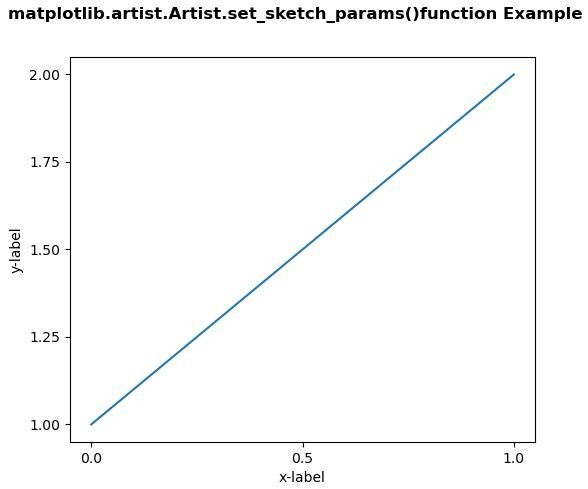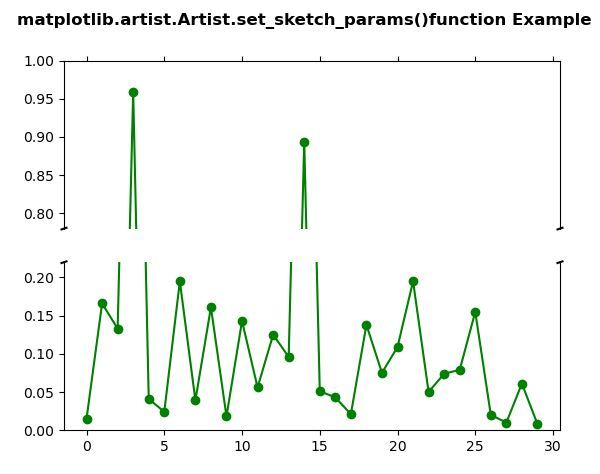# Matplotlib.artist.Artist.set_sketch_params() in Python

• Last Updated : 10 May, 2020

Matplotlib is a library in Python and it is numerical – mathematical extension for NumPy library. The Artist class contains Abstract base class for objects that render into a FigureCanvas. All visible elements in a figure are subclasses of Artist.

## matplotlib.artist.Artist.set_sketch_params() method

Attention geek! Strengthen your foundations with the Python Programming Foundation Course and learn the basics.

To begin with, your interview preparations Enhance your Data Structures concepts with the Python DS Course. And to begin with your Machine Learning Journey, join the Machine Learning - Basic Level Course

The set_sketch_params() method in artist module of matplotlib library is used to sets the sketch parameters.

Syntax: Artist.set_sketch_params(self, scale=None, length=None, randomness=None)

Parameters: This method accepts the following parameters.

• scale: This parameter is the amplitude of the wiggle perpendicular to the source line, in pixels.
• length: This parameter is the length of the wiggle along the line, in pixels.
• randomness : This parameter is the scale factor by which the length is shrunken or expanded.

Returns: This method does not return any value.

Below examples illustrate the matplotlib.artist.Artist.set_sketch_params() function in matplotlib:

Example 1:

 `# Implementation of matplotlib function``from` `matplotlib.artist ``import` `Artist  ``import` `matplotlib.pyplot as plt ``import` `matplotlib.colors as mcolors ``import` `matplotlib.gridspec as gridspec ``import` `numpy as np ``     ` `     ` `plt.rcParams[``'savefig.facecolor'``] ``=` `"0.8"``plt.rcParams[``'figure.figsize'``] ``=` `6``, ``5``     ` `fig, ax ``=` `plt.subplots() ``     ` `ax.plot([``1``, ``2``]) ``     ` `ax.locator_params(``"x"``, nbins ``=` `3``) ``ax.locator_params(``"y"``, nbins ``=` `5``) ``     ` `ax.set_xlabel(``'x-label'``) ``ax.set_ylabel(``'y-label'``) ``   ` `Artist.set_sketch_params(ax, ``100``, ``100``, ``20``) `` ` `fig.suptitle('matplotlib.artist.Artist.set_sketch_params()\``function Example', fontweight ``=``"bold"``) `` ` `plt.show()`

Output:Example 2:

 `# Implementation of matplotlib function``from` `matplotlib.artist ``import` `Artist  ``import` `matplotlib.pyplot as plt ``import` `numpy as np ``    ` `values ``=` `np.array([ ``    ``0.015``, ``0.166``, ``0.133``,  ``    ``0.159``, ``0.041``, ``0.024``, ``    ``0.195``, ``0.039``, ``0.161``, ``    ``0.018``, ``0.143``, ``0.056``, ``    ``0.125``, ``0.096``, ``0.094``, ``    ``0.051``, ``0.043``, ``0.021``, ``    ``0.138``, ``0.075``, ``0.109``, ``    ``0.195``, ``0.050``, ``0.074``,  ``    ``0.079``, ``0.155``, ``0.020``, ``    ``0.010``, ``0.061``, ``0.008``]) ``    ` `values[[``3``, ``14``]] ``+``=` `.``8``    ` `fig, (ax, ax2) ``=` `plt.subplots(``2``, ``1``, sharex ``=` `True``) ``    ` `ax.plot(values, ``"o-"``, color ``=``"green"``) ``ax2.plot(values, ``"o-"``, color ``=``"green"``) ``    ` `ax.set_ylim(.``78``, ``1.``)  ``ax2.set_ylim(``0``, .``22``) ``    ` `ax.spines[``'bottom'``].set_visible(``False``) ``ax2.spines[``'top'``].set_visible(``False``) ``ax.xaxis.tick_top() ``ax.tick_params(labeltop ``=` `False``) ``ax2.xaxis.tick_bottom() ``    ` `d ``=` `.``005``kwargs ``=` `dict``(transform ``=` `ax.transAxes,  ``              ``color ``=``'k'``, clip_on ``=` `False``) ``   ` `ax.plot((``-``d, ``+``d), (``-``d, ``+``d), ``*``*``kwargs)        ``ax.plot((``1` `-` `d, ``1` `+` `d), (``-``d, ``+``d), ``*``*``kwargs)  ``    ` `kwargs.update(transform ``=` `ax2.transAxes)   ``   ` `ax2.plot((``-``d, ``+``d), (``1` `-` `d, ``1` `+` `d), ``*``*``kwargs) ``ax2.plot((``1` `-` `d, ``1` `+` `d), (``1` `-` `d, ``1` `+` `d), ``*``*``kwargs)  `` ` `Artist.set_sketch_params(ax, ``1.0``, ``100.0``, ``22.0``) ``Artist.set_sketch_params(ax2, ``1.0``, ``10.0``, ``22.0``)`` ` `fig.suptitle('matplotlib.artist.Artist.set_sketch_params()\``function Example', fontweight ``=``"bold"``) `` ` `plt.show()`

Output:My Personal Notes arrow_drop_up